# Phase Diagram Module

## Constructing a Blast Furnace Diagram with FactSage 8.2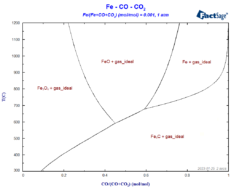The so-called blast-furnace diagram describes which phases are stable in the different regions of the blast furnace, depending on the conditions of temperature and of the atmosphere inside the furnace. It is basically a phase diagram, where the volume fraction of CO is represented on the X-axis and the temperature is represented on the Y-axis, …

## 3D Mapping of Gibbs Energy Diagrams onto Phase Diagrams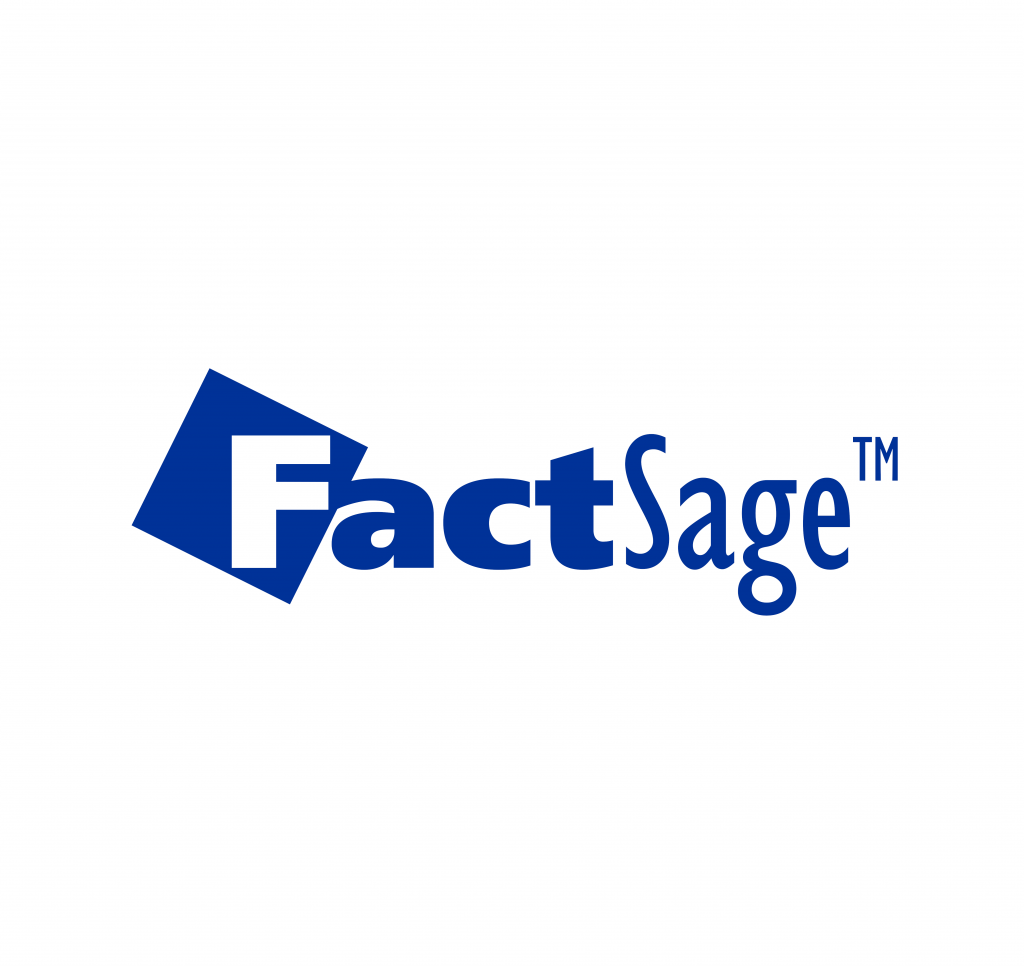Mats Hillert has shown [*] that what is called a Phase Diagram is derivable from a projection in a so-called Property Diagram. The (molar) Gibbs Energy as the property is plotted along the z-axis as a function of two other variables x and y. For binary systems, for example, T equals y and the mole …

## From molar Gibbs energy functions to phase diagrams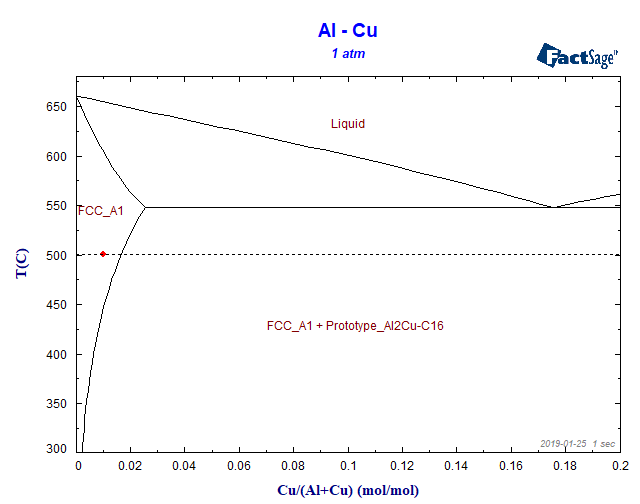In a series of posts, we will focus on the relation between phase diagrams, Gibbs energy functions and activities. Let us begin with the Al-Cu phase diagram at a temperature of 500 °C. In this blog post we will work exclusively with the FactSage Education Package. Let us suppose we have generated an Al-Cu phase-diagram …

## Example for a FactSage calculation of a non-equilibrium process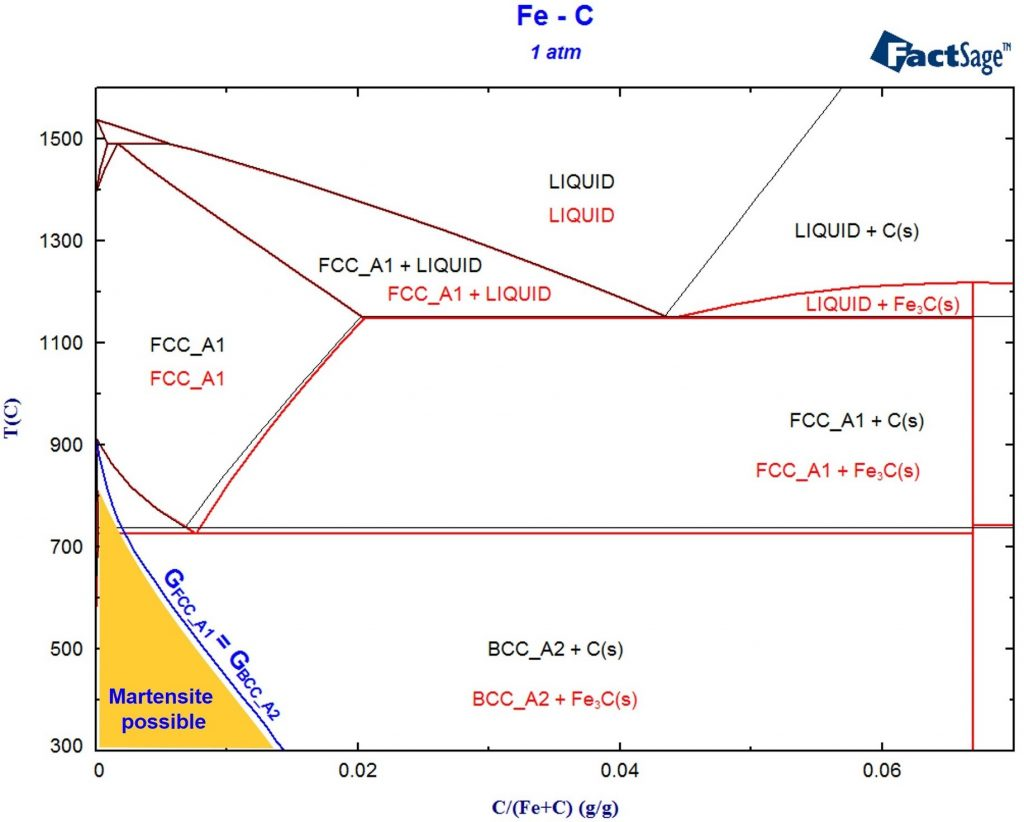It is a common misconception that thermodynamic calculations can only be used for processes that are in equilibrium. In fact, there is a lot to learn from an Integrated Thermodynamic Databank System like FactSage even if you are far away from equilibrium! As example we consider the popular Fe-C system: If you choose all phases …

## A hands-on example for a phase-diagram-module calculation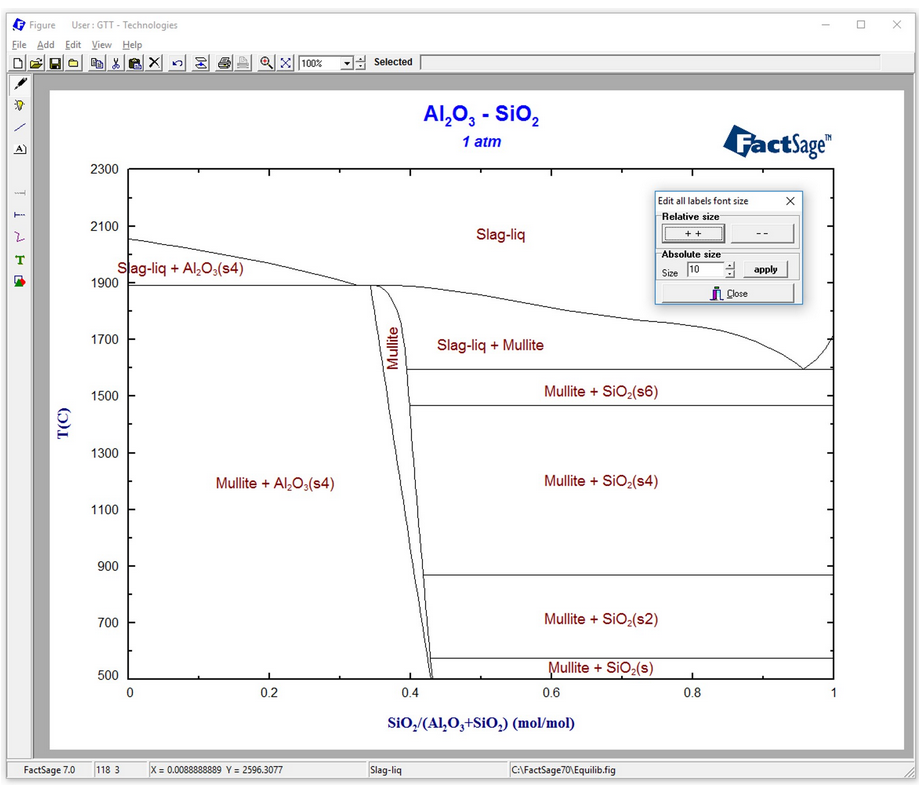FactSage contains many modules. The major ones for daily work are Equilib and Phase Diagram. Phase Diagram permits the calculation of phase diagram sections for systems with any number of components. Components can be elements, compounds or even arbitrary compositions. For a phase diagram calculation, first the components of interest have to be defined. Then, the possible …